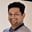Related Tags

java
communitycreator

# How to solve the Happy Number problem in JavaTarun Telang

### Problem description

In this shot, we will solve an interview problem from Leetcode called Happy Number.

We will learn how to determine whether or not a number is happy. A happy number is a number that converges to a sum of $1$ after getting replaced by the sum of the square of its digits.

### Examples

Let’s understand the Happy Number problem with the help of examples:

1. Suppose the number is $44$. We have the sum of squares of digits as below:

$4^2$ + $4^2$ = 16 + 16 = 32

$3^2$ + $2^2$ = 9 + 4 = 13

$3^2$ + $1^2$ = 9 + 1 = 10

$1^2$ + $0^2$ = 1 + 0 = 1

Finally, the sum of squares of the digits converges to 1, so 44 is a happy number. The program’s output for this input would be true.

1. Now, suppose the number is 16, here we have the sum of squares of digits as below:

$1^2$ + $6^2$ = 1 + 36 = 37

$3^2$ + $7^2$ = 9 + 49 = 58

$5^2$ + $9^2$ = 25 + 49 = 74

$7^2$ + $4^2$ = 49 + 16 = 65

$6^2$ + $5^2$ = 36 + 25 = 61

$6^2$ + $1^2$ = 36 + 1 = 37

Here the sum of the digits’ squares is not converging to $37$, so it would keep repeating with the pattern (37, 58, 74, 65, 61, 37, 58, 74, … so on). Therefore, $16$ is not a happy number, and the program’s output for this input would be false.

### Solution approach

We first find the sum of digits using a while loop and the modulo % operator. Then, we will store the values within a java.util.Set to check whether or not the sum value has been encountered previously.

The code below provides a solution to the Happy Number problem:

import java.util.*;

class Solution {
public static boolean isHappy(int n) {
Set<Integer> set = new HashSet<>();

while (n!=1) {
if(set.contains(n)) return false;

n = sumOfDigitSquares(n);
}

return true;
}

private static int sumOfDigitSquares(int n) {
int sum = 0;
while (n != 0) {
int digit = n % 10;
sum += digit * digit;
n /= 10;
}

return sum;
}

public static void main(String[] args) {
System.out.printf("Is 44 happy -> %b", isHappy(44));
System.out.printf("\nIs 16 happy -> %b", isHappy(16));
}
}
Java program to solve the Happy Number problem

### Explanation

• Line 1 imports java.util.* (using a wildcard as we want to import both Set and HashSet).

• Line 3 declares a Solution class.

• Line 4 defines the isHappy(int n) method.

• Line 5 declares a set using java.util.HashSet to store integers.

• Line 7 repeats a while loop until the value of n equals 1.

• Line 8 checks if the value of n is already present in Set. If so, it returns false denoting the number n is not a happy number.

• Line 10 adds n to the set.

• Line 11 calculates the sum of the square of digits using the sumOfDigitSquares() method and updates the value of n with the returned value.

• Line 12 exits the while loop when n == 1.

• Line 14 returns true, denoting the number as a happy number.

• Line 17 declares the sumOfDigitSquares(int n) method.

• Line 18 initializes sum to 0.

• Line 19 repeats a while loop until n is not equal to 0.

• Line 20 gets the current digit as n % 10.

• Line 21 updates the sum as the current value plus square of digit (i.e. digit * digit).

• Line 22 updates n to n / 10 (integer division).

• Lines 23 and 25 exit the while loop if n equals 0 and return sum.

• Line 28 declares the main() method to verify our solution.

• Lines 29 and 30 call the isHappy() with different inputs and print the output.

### Analysis

The time complexity of this problem would be $O(d)$, where $d$ is the number of digits in the number input. The max value of $d$ can be $10$, as in Java, the range of integers is up to $2,147,483,647$ (which has $10$ digits). More precisely, the algorithm runs in constant time $O(1)$.

As the memory required for this program does not depend on the input value, the space complexity of this problem would be constant, i.e., $O(1)$.

RELATED TAGS

java
communitycreator

CONTRIBUTORTarun Telang
RELATED COURSES

View all Courses

Keep Exploring

Learn in-demand tech skills in half the time